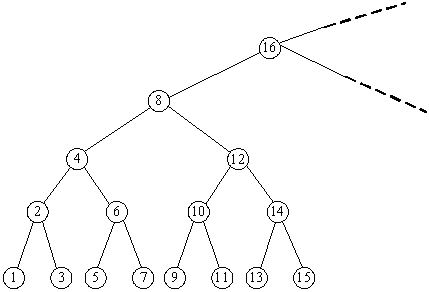# Competitive Programming: POJ 1654 – Area

### Description

Consider an infinite full binary search tree (see the figure below), the numbers in the nodes are 1, 2, 3, …. In a subtree whose root node is X, we can get the minimum number in this subtree by repeating going down the left node until the last level, and we can also find the maximum number by going down the right node. Now you are given some queries as “What are the minimum and maximum numbers in the subtree whose root node is X?” Please try to find answers for the queries.### Input

In the input, the first line contains an integer N, which represents the number of queries. In the next N lines, each contains a number representing a subtree with root number X (1 <= X <= 231 – 1).

### Output

There are N lines in total, the i-th of which contains the answer for the i-th query.

```2
8
10
```

### Sample Output

```1 15
9 11
```
```import java.util.Scanner;

/*
There's a pattern with the lowest-order set bit of the numbers:
- For leaf-node numbers, the lowest order set bit is 1.
- Go up one level and the lowest order set bit is 2.
- Go up one more level and the lowest order set bit is 4.
- Etc.

That said, we can take advantage of Bit Hack #7 from
http://www.catonmat.net/blog/low-level-bit-hacks-you-absolutely-must-know/
to isolate the lowest-order set bit.

Each node will have one less than that number of nodes in each subtree.
*/

public class Main {

public static void main(String[] args) {
Scanner sc = new Scanner(System.in);

int N = sc.nextInt();
while (N-- > 0) {
int x = sc.nextInt();
int diff = (x & (-x)) -1;
System.out.println((x - diff) + " " + (x + diff));
}

sc.close();
}
}
```